# Two machines

Performances of two machines are in a ratio of 7:12. A machine with less power produced 406 pieces of products per shift.

a) How many pieces produced per shift second machine?
b) How many pieces produced two machines together for five shifts?

a =  696
b =  5510

### Step-by-step explanation: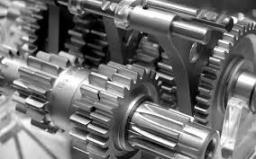Did you find an error or inaccuracy? Feel free to write us. Thank you!Tips to related online calculators
Check out our ratio calculator.

## Related math problems and questions:

• Two xeroxes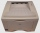The performances of the two copiers are in the ratio 3: 4. A machine with higher power will make 7,200 copies in one hour. How many copies will both machines make together in 5 hours?
• Evaluate expressionEvaluate following expression: 5 to the power of 2 - (4x4) divided by 2 =
• Variability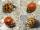In the table bellow the number of wrong produced goods in two shifts: morning shift: 2; 0; 6; 10; 2; 2; 4; 2; 5; 2; afternoon shift: 4; 4; 0; 2; 10; 2; 6; 2; 3; 10; Compare the variability in both shifts, compare the average number of wrong goods on both
• The cubeThe cube has a surface area of 486 m ^ 2. Calculate its volume.
• Machines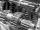In the workshop are three machines. The first is treated 250 parts for 4.7 hours, the second is treated 300 parts for 1.9 hours, and the third is treated 230 parts for 4.6 hours. How long will it take to make 3100 parts if worked all three machines at the
• Sugar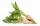From 3 tons of sugar beet was produced 480 kg of sugar. How many tons of sugar was produced from 17.5 tons of sugar beet?
• How manyHow many numbers are less than 222 with a digit sum is 8?
• Expression with bracketsEvaluate the expression. 15+[(9-4)×22]
• Moneys in triple ratioMilan, John and Lili have a total 344 euros. Their amounts are in the ratio 1:2:5. Determine how much each of them has?
• Volume per timeHow long does fill take for a pump with a volume flow of 200 l per minute fill a cube-shaped tank up to 75% of its height if the length of the cube edge is 4 m?
• Ratio6 numbers are in the ratio 1:5:1:5:5:5. Their sum is 242. What are the numbers?
• Natural gas in kWh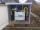Gas consumption for 2017 was 11,301 kWh I paid 532 € How much 1 m3?
• PointsGryffindor won 437 points. How many points obtained by each of the faculties if they were split at a ratio of 5: 7: 3: 4?
• Machines 2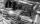2 machines for 50 hours produces 2,000 products. How many machines need to buy to make in 30 hours 15,000 products?
• The cubeThe cube has a surface of 600 cm2. What is its volume?
• Evaluate 9Evaluate expression: 3 { 4 − 4 ( 5 − 12 ) } + 20
• TogetherThe three friends divided the balls in a ratio of 6: 5: 4. Some two of them got a total of 126 balls. How many balls were there together?